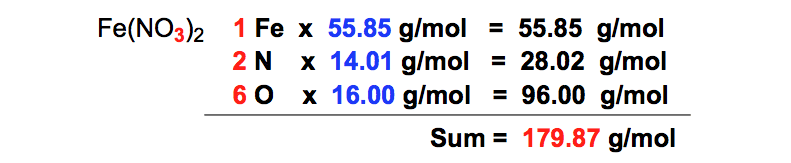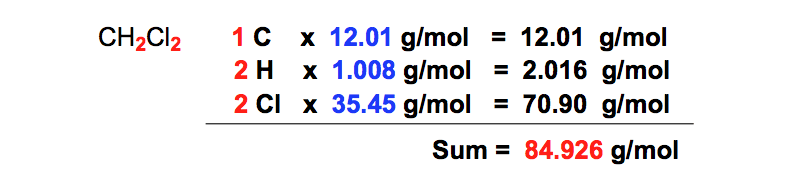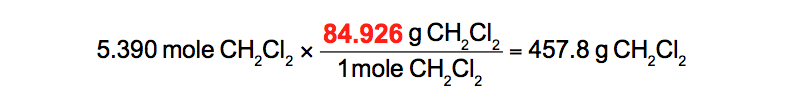Ch.2 - Atoms & ElementsWorksheetSee all chapters
 Ch.1 - Intro to General Chemistry 2hrs & 53mins 0% complete Worksheet Ch.2 - Atoms & Elements 2hrs & 40mins 0% complete Worksheet Ch.3 - Chemical Reactions 3hrs & 25mins 0% complete Worksheet BONUS: Lab Techniques and Procedures 1hr & 38mins 0% complete Worksheet BONUS: Mathematical Operations and Functions 47mins 0% complete Worksheet Ch.4 - Chemical Quantities & Aqueous Reactions 3hrs & 30mins 0% complete Worksheet Ch.5 - Gases 3hrs & 47mins 0% complete Worksheet Ch.6 - Thermochemistry 2hrs & 28mins 0% complete Worksheet Ch.7 - Quantum Mechanics 2hrs & 35mins 0% complete Worksheet Ch.8 - Periodic Properties of the Elements 1hr & 57mins 0% complete Worksheet Ch.9 - Bonding & Molecular Structure 2hrs & 5mins 0% complete Worksheet Ch.10 - Molecular Shapes & Valence Bond Theory 1hr & 31mins 0% complete Worksheet Ch.11 - Liquids, Solids & Intermolecular Forces 3hrs & 40mins 0% complete Worksheet Ch.12 - Solutions 2hrs & 17mins 0% complete Worksheet Ch.13 - Chemical Kinetics 2hrs & 22mins 0% complete Worksheet Ch.14 - Chemical Equilibrium 2hrs & 26mins 0% complete Worksheet Ch.15 - Acid and Base Equilibrium 4hrs & 42mins 0% complete Worksheet Ch.16 - Aqueous Equilibrium 3hrs & 48mins 0% complete Worksheet Ch. 17 - Chemical Thermodynamics 1hr & 44mins 0% complete Worksheet Ch.18 - Electrochemistry 2hrs & 58mins 0% complete Worksheet Ch.19 - Nuclear Chemistry 1hr & 33mins 0% complete Worksheet Ch.20 - Organic Chemistry 3hrs 0% complete Worksheet Ch.22 - Chemistry of the Nonmetals 2hrs & 1min 0% complete Worksheet Ch.23 - Transition Metals and Coordination Compounds 1hr & 54mins 0% complete Worksheet

# Calculating Grams to Moles

See all sections
Sections
Periodic Table
Mole Concept
Isotopes
Subatomic Particles
Atomic Theory
Thomson's Cathode Ray Tube Experiment
Oil Drop Experiment
Gold Foil Experiment
Mass SpectrometryJules Bruno

Molar mass or molecular weight is a physical property that represents the mass of a substance divided by the amount of that substance. It serves as the bridge for the conversion between the grams and moles of a substance.

How to Calculate Molar Mass

To calculate the molar mass of a compound we must determine the number of atoms for the elements within a given compound. The common units are typically in grams per mole (g/mol).

For example, if we are asked to determine the molar mass of iron (II) nitrate, Fe(NO3)2, we can solve with steps shown below.

STEP 1: Count the number of each element within the compound that contains 1 iron, 2 nitrogens and 6 oxygens.Chemical Formula (Counting Atoms)

Realize that the NO3 group in parentheses has the “2” distributed to the nitrogen and oxygen.

STEP 2: Find the atomic mass for each chemical element from the periodic table.Atomic Masses

STEP 3: Multiply the number of each element by their atomic masses taken from the periodic table.Calculating masses

STEP 4: Add up all the masses, obtained from multiplication, together to give the overall molar mass of the compound.Molar Mass of Iron (II) Nitrate

Once you know the molar mass or molecular weight of a compound you can convert between grams and moles.

How to convert from grams to moles

To convert from grams to moles we must divide the given gram amount by the molar mass of the compound.

For example, if we are asked "how many moles are there in a 21.3 g Fe(NO3)2 sample?", this is how we should setup our problem:Grams to Moles Conversion

How to convert from moles to grams

Now what do we do when are given the moles of a compound and asked to determine its mass in grams?

For example, if we are asked, "how many grams are there in a 5.390 mole CH2Clsample", this is how we should begin:

STEP 1: Count the number of each element within the compound that contains 1 carbon, 2 hydrogens and 2 chlorines.Chemical Formula (Counting atoms)

STEP 2: Find the atomic mass for each chemical element from the periodic table.Atomic Masses of Carbon, Hydrogen and Chlorine

STEP 3: Add up all the masses, obtained from multiplication, together to give the overall molar mass of the compound.Calculating molar mass (CH2Cl2)

STEP 3: Multiply the given mole amount by the molar mass of the compound.Moles to grams conversion

Beyond mass conversions

Mass conversions are a fundamental process in chemistry that will be used through numerous chapters. This concept will connect us to others such as molaritystoichiometry, and solution chemistry where we deal with the concentration of homogeneous mixtures.Jules Bruno

Jules felt a void in his life after his English degree from Duke, so he started tutoring in 2007 and got a B.S. in Chemistry from FIU. He’s exceptionally skilled at making concepts dead simple and helping students in covalent bonds of knowledge.